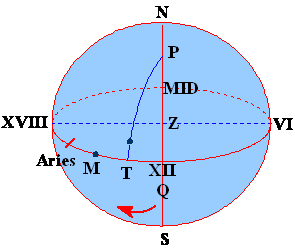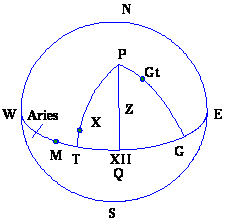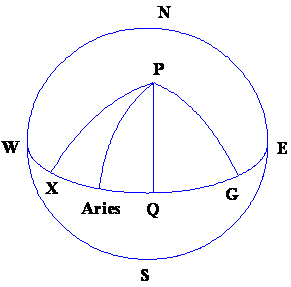Back to Nautical Solar Sys. Hour Angles PZX Triangles Dip Amplitude Day Ex Meridian Exercises Pole Star Naut. Almanac

Day

The Earth rotates on its axis, and due to this rotation the sun appears over a particular meridian after each rotation of the earth. The time interval between two successive passage of the sun over the same meridian is called a SOLAR DAY.

The time period between two successive passes of the Moon over the same meridian is called a LUNAR DAY.

And the time period between two successive passes of the ‘First Point Aries’ is called a SIDEREAL DAY.

The earth’s motion is not uniform thus the motion of the sun appears also to be erratic. The transits of the sun over the meridian which bring about the time interval called day is thus not uniform. The TRUE SUN therefore registers what is called the APPARENT SOLAR TIME or APPARENT SOLAR DAY.

Since we cannot work with a non uniform rotation a mean is used, thus the mean rotation of the Earth over the entire year is taken and the time interval that is obtained is called the MEAN SOLAR DAY. This is equal in length to the mean or average of all the apparent solar days in the year.

Since the ‘First Point of Aries’, an imaginary point is fixed, thus the Sidereal Day is constant. The Lunar Day is longer than the Solar Day and the Solar Day is longer than the Sidereal Day.

The Local Hour Angle of a body is its angular distance WEST of the observer’s meridian expressed in unit of time from 0h to 24h or in the unit of angular measurement from 0˚ to 360˚.

The LHA is expressed either as an arc of the celestial equator or by the angle at the pole conatained between the observers meridian and the meridian of the body.

The LHA subtracted from 24h would give the EAST Hour Angle.

NOTE: When only Hour Angle is mentioned it is meant as WEST Hour Angle.

Right Ascension of a body:

Is its angular distance EAST of the first point of Aries expressed in hours from 0h to 24h, it is the arc of the celestial equator between Aries and the meridian passing through the body.

Ship Mean Time: or Local Mean Time: is the local hour angle of the mean sun (LHA mean sun) + 12 h, and is represented by the arc Mid VI, QM on our figs, or simply, arc MQ+12h

Suppose PT were the meridian passing through the true sun, then the arc QT would be the local hour angle true sun (LHA sun) and this quantity which increases as the sun moves WESTWARD is found from direct sextant and time observation on board ship.SHIP’S APPARENT TIME (SAT) or LOCAL APPARENT TIME (LAT):

Lat IS THE local Hour Angle of the true sun + 12 hours

Or LHA (sun) + 12 hours

In the above figure it is the arc MID – VI – QT

Equation of Time

This is a quantity, which connects the Mean and the Apparent times. The true sun is sometimes ahead or sometimes behind the average or the Mean sun, and the Equation is the time interval which separates them.

In the above figure,

Arc (QM+12h) – arc (QT+12h) = arc MT, the equation of Time

It is subtracted from or added to, the apparent time to get the corresponding mean time, as tabulated in the Almanac.

The EQ. Of Time would be plus to apparent time for the relative position of M and T as shown in the fig. Because the mean sun is ahead of the true sun.